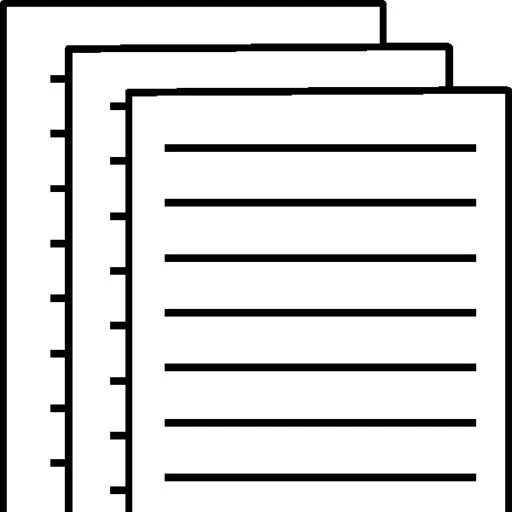## AB 204 Unit 9 Assignment

AB204-02 Unit 9 Assignment

Kaplan University

May 02,2017

Unit 9Assignment

Refer to the sets of the aggregate demand, short-run aggregate supply, and long-run aggregate supply curves. Use the graphs to explain the process and steps by which each of the following economic scenarios will shift the economy from one long-run macroeconomic equilibrium to another equilibrium. Under each scenario, elaborate the short-run and long-run effects of the shifts in the aggregate demand and aggregate supply curves on the aggregate price level and aggregate output (real GDP).

Suppose the household wealth decreases due to a decline in the stock market asset prices (See the set of graphs below and pay attention to the 3-stage shifts in graphs).

Suppose the economy is in long run equilibrium first. That is, the long run aggregate supply curve, the short run aggregate supply curve, and the aggregate demand curve, they all intersect at the current level of output. Now, the household wealth decreases due to a decline in the stock market asset prices. This means the households will reduce their consumption spending. This will lead the AD curve to shift leftward. The new intersection of the AD and SRAS curve will occur at a lower price level and an output level below the natural level. Since the output is below the natural level, the price will continue to decline, implying that the aggregate supply curve will continue to shift rightward so as to restore the output level back to the natural level. At the new long run equilibrium, the three curves again intersect, but now at a price level lower than before, but the output level same as before.

b. Assume the government lowers taxes, which increases the household’s disposable income. However, the government purchases (spending) remains the same. (See the set of graphs below and shifts in graphs)

Suppose the economy is in long run equilibrium first. That is, the long run aggregate supply curve, the short run aggregate supply curve, and the aggregate demand curve, they all intersect at the current level of output. Now, the government decreases taxes. This means the households’ disposable income will increase, implying that consumer spending will rise. This will lead the AD curve to shift rightward. The new intersection of the AD and SRAS curve will occur at a higher price level and an output level above the natural level. Since the output is above the natural level, the price will continue to rise, implying that the aggregate supply curve will continue to shift leftward so as to restore the output level back to the natural level. At the new long run equilibrium, the three curves again intersect, but now at a price level higher than before, but the output level same as before.

2. Suppose the economy of a hypothetical country has reached its long-run macroeconomic equilibrium when each of the following aggregate demand shocks occurs. What kind of gap, inflationary or recessionary gap, will the economy face after the AD shock indicated by the shift in AD curves? What types of fiscal policy instruments will help move the economy back to the potential level of output (real GDP)? Give specific examples.

a. At the long-run macroeconomic equilibrium, the stock market boom occurs and this increases the value of stocks households hold. (See the set of graphs below and shifts in graphs in the two-steps)

When the stock market booms, the value of stocks held by households rises. Consumer spending will increase, and the aggregate demand curve will shift to the right. This is considered an inflationary gap. Contractionary fiscal policies could move the economy back to potential level of output.

b. The government increases its purchases (spending) due to natural disasters. (See the set of graphs below and shifts in graphs)

As government spending increases, the aggregate demand curve shifts to the right. This is considered an inflationary gap. Contractionary fiscal policies could move the economy back to potential level of output, but the government would have to cut back on spending, increase taxes, or reduce transfers.

Assume the Central Bank reduces the money supply in the economy which leads to an increase in the interest rates. (See the set of graphs below and shifts in graphs)

As Central Bank reduces the money supply in the economy and interest rates rise, investment spending decreases and the aggregate demand will shift to the left. This is considered a recessionary gap. Expansionary fiscal policies could move the economy to potential level output.

References

Mankiw, N. G. (2015). Principles of Macroeconomics, 7th Edition. [Kaplan]. Retrieved from https://kaplan.vitalsource.com/#/books/9781305156067/https://kaplan.vitalsource.com/#/books/9781305156067/

Mankiw, N. G. (2015). Principles of Macroeconomics, 7th Edition. [Kaplan]. Retrieved from https://kaplan.vitalsource.com/#/books/9781305156067/

To view and download a complete answer, scroll down to the bottom to pay